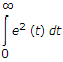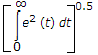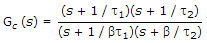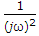# Electronics and Communication Engineering - Automatic Control Systems

Exercise : Automatic Control Systems - Section 1
31.
If error voltage is e(t), integral square error =
e2(t) dtnone of the above
Explanation:

Error is squared and integrated.

32.
A lag compensator is essentially a
low pass filter
high pass filter
band pass filter
either (a) or (b)
Explanation:

See transfer function in the equation, β > 1

where t1 = R1C1, t2 = R2C2 and t > 1.

33.
Stepper motors find applications in
X-Y plotters
numerically controlled machining equipment
printers
all of the above
Explanation:

Stepper motors are used in all these applications.

34.
For type 2 system, the magnitude and phase angle of the term (jω)2 in the denominator, at ω = 0, are respectively
0 and - 90°
0 and + 90°
infinity and - 180°
infinity and + 180°
Explanation:

If ω = 0,has a magnitude of infinity and phase angle -180°.

35.
In an integral controller
the output is proportional to input
the rate of change of output is proportional to input
the output is proportional to rate of change of input
none of the above